#0
0
01. 云栖社区>
2. 博客>
3. 正文

## 数据结构（十）：最小生成树为最小生成树的一个真子集，即的顶点集合和边集合都是的顶点和边集合的子集，构造最小生成树过程为向中添加顶点和边，添加的原则有两种：

1. 选择的边集合外，权值最小的边，加入到1. 选择的顶点集合外，距离最近的顶点，加入到### kruskal 算法

`kruskal` 算法即为上述第一种原则，通过选择图中的最小权值边来构造最小生成树，过程中需要注意避免形成环。

##### 算法过程
1. 对边集合进行排序
2. 选择最小权值边，若不构成环，则添加到集合3. 重复执行步骤 2，直到添加条边
##### 演示示例graph

step 1：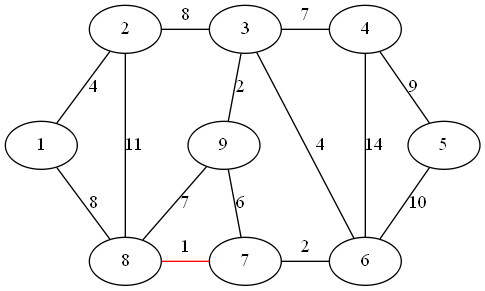step 2：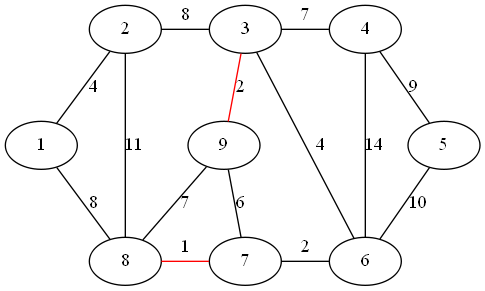step 3：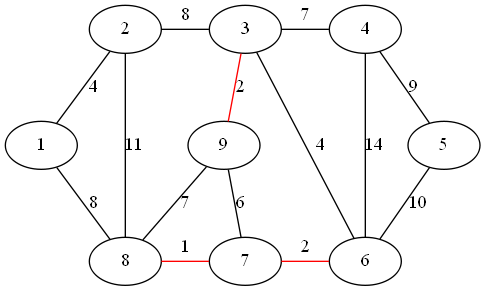step 4：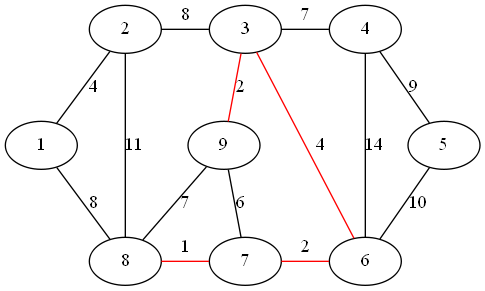step 5：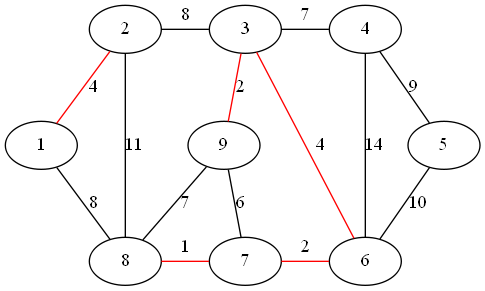step 6：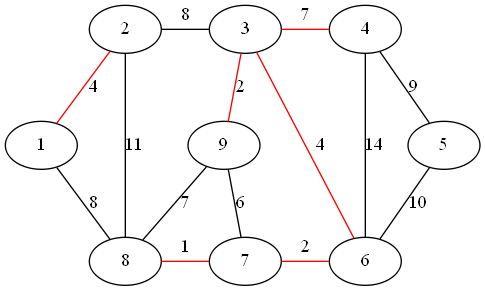step 7：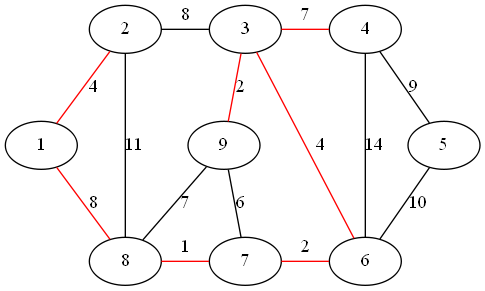step 8：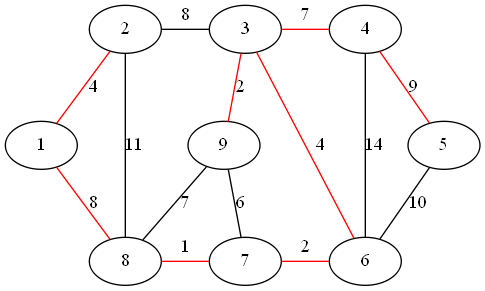##### 算法示例

1. `kruskal` 算法示例
``````def kruskal(graph):
edges, vertices = getEdgesFromAdjacencyList(graph), [i for i in range(graph.number)]
sort(edges, 0, len(edges) - 1)
weightSum, edgeNumber = 0, 0
while edgeNumber < graph.number - 1:
edge = edges.pop()
beginOrigin, endOrigin = origin(vertices, edge.begin - 1), origin(vertices, edge.end - 1)
if (beginOrigin != endOrigin): # whether the two vertices belong to same graph
vertices[beginOrigin] = endOrigin  # identify the two vertices in the same sub graph
weightSum, edgeNumber = weightSum + edge.weight, edgeNumber + 1  # calculate the total weight
``````

`kruskal` 算法设定最初每个顶点都是一个子图，每个子图都有一个根，或者称之为出发点，每个加入的顶点都保留一个指向上一个顶点的引用，并最终追溯到该子图的根顶点，所以可以通过判断两个顶点指向的根顶点是否相同，来判断两顶点是否属于同一个子图。

1. 邻接表转边集合
``````def getEdgesFromAdjacencyList(graph):
edges = []
for i in range(graph.number):
node = graph.list[i]
while node:
edge, node = Edge(i + 1, node.index, node.weight), node.next
edges.append(edge)
return edges
``````

1. 判断两个顶点是否属于同一个子图，避免添加边后形成环
``````def origin(vertices, index):
while vertices[index] != index:
index = vertices[index]
return index
``````

##### 性能分析

`kruskal` 算法中使用 `getEdgesFromAdjacencyList` 函数完成邻接表向边集合的转换，函数内部存在两层循环，访问邻接表中每个顶点的相邻顶点，复杂度为。使用快排对边集合进行排序，时间复杂度为，因为，所以快排时间复杂度可以表述为`kruskal` 算法中 `while` 循环取最小权值边，并对边的两个顶点执行 `origin` 函数判断是否属于同一个子图，时间复杂度为。所以 `kruskal` 算法的时间复杂度为### prim 算法

`kruskal` 算法的过程为不断对子图进行合并，直到形成最终的最小生成树。`prim` 算法的过程则是只存在一个子图，不断选择顶点加入到该子图中，即通过对子图进行扩张，直到形成最终的最小生成树。

##### 算法过程
1. 按照距离子图的远近，对顶点集合进行排序
2. 选择最近的顶点加入到子图中，并更新相邻顶点对子图的距离
3. 重复执行步骤 2，直到顶点集合为空
##### 演示示例graph

step 1：step 2：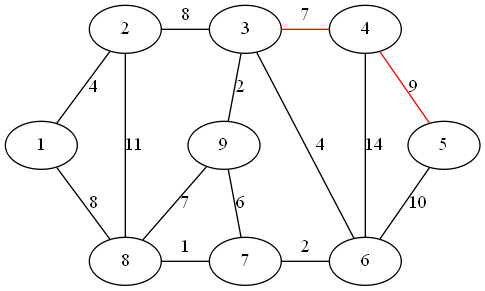step 3：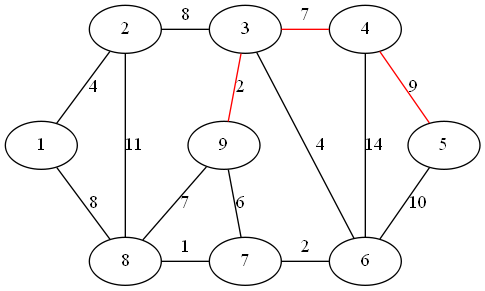step 4：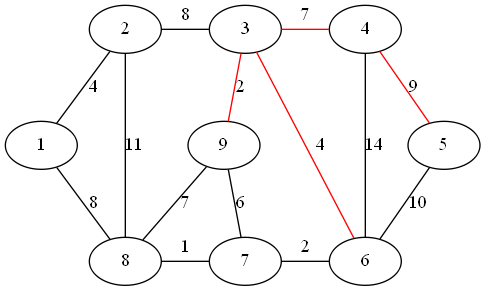step 5：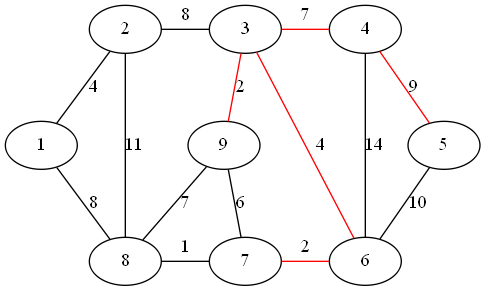step 6：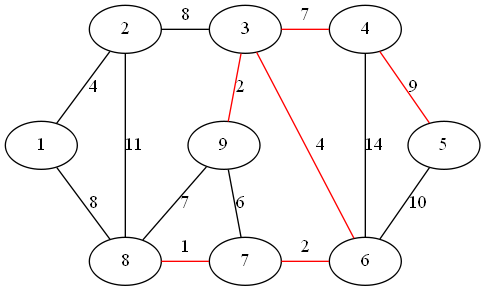step 7：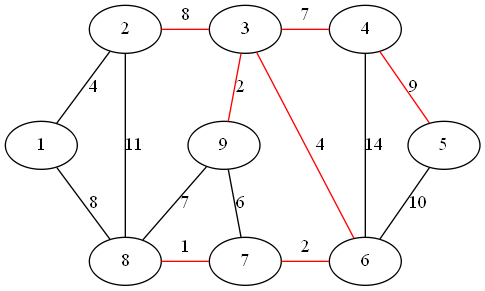step 8：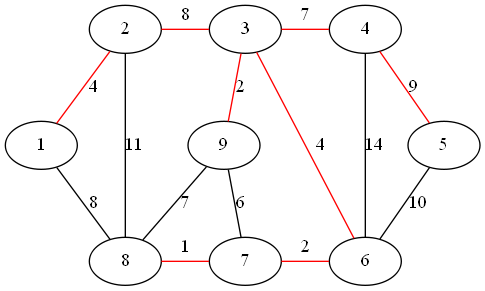##### 算法示例

1. `prim` 算法示例
``````def prim(graph, index):
vertices, verticesIndex = [{'index': i, 'weight': None} for i in range(graph.number)], [i for i in range(graph.number)]
weightSum, vertices[index - 1]['weight'] = 0, 0
heapSort(vertices, verticesIndex)
while len(vertices) > 0:
swapVertices(vertices, verticesIndex, 0, -1)
vertex = vertices.pop()
transformToHeap(vertices, verticesIndex, 0, len(vertices))
weightSum = weightSum + vertex['weight']
updateVertices(graph, vertices, verticesIndex, vertex['index'])
``````

1. 交换堆顶元素
``````def swapVertices(vertices, verticesIndex, origin, target):
vertices[origin], vertices[target] = vertices[target], vertices[origin]
verticesIndex[vertices[origin]['index']], verticesIndex[vertices[target]['index']] = origin, target
``````

`vertices` 列表调整为小顶堆之后，将列表首、尾元素交换，则列表尾元素即为距离子图最近的顶点元素。

1. 添加顶点到子图中后，更新相邻顶点到子图的距离
``````def updateVertices(graph, vertices, verticesIndex, index):
node = graph.list[index]
while node:
if verticesIndex[node.index - 1] == -1:
node = node.next
continue
vertex = vertices[verticesIndex[node.index - 1]]
if not vertex['weight'] or (vertex['weight'] and vertex['weight'] > node.weight):
vertex['weight'] = node.weight
pos = verticesIndex[vertex['index']]
while pos > 0 and (not vertices[(pos - 1) // 2]['weight'] or vertices[pos]['weight'] < vertices[(pos - 1) // 2]['weight']):
swapVertices(vertices, verticesIndex, pos, (pos - 1) // 2)
pos = (pos - 1) // 2
node = node.next
``````

##### 性能分析

`prim` 算法中构造顶点列表的时间复杂度为。使用堆排序对顶点列表进行排序，时间复杂度为`prim` 算法中 `while` 循环取最近顶点元素，并调整元素取出后列表的堆结构，所以总体的调整复杂度为；同时循环结构内执行 `updateVertices` 函数，更新每个取出顶点的相邻顶点距离值，所以总体的更新顶点数为，因为每个顶点更新距离后，需要调整堆结构为小顶堆，所以总体的复杂度为。所以`prim` 算法的时间复杂度为+ 关注